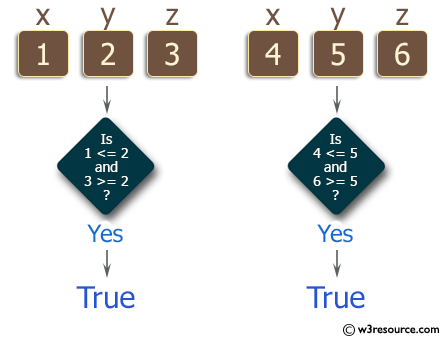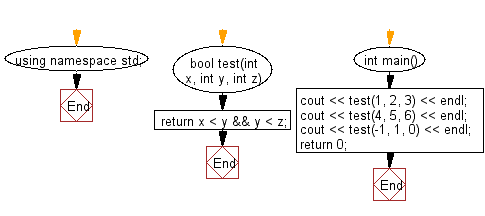﻿ C++ : Check if y is greater than x, and z is greater than y# C++ Exercises: Check if y is greater than x, and z is greater than y from three given integers x,y,z

## C++ Basic Algorithm: Exercise-45 with Solution

Write a C++ program to check if y is greater than x, and z is greater than y from three given integers x,y,z.

Sample Solution:

C++ Code :

``````#include <iostream>

using namespace std;

bool test(int x, int y, int z)
{
return x < y && y < z;
}

int main()
{
cout << test(1, 2, 3) << endl;
cout << test(4, 5, 6) << endl;
cout << test(-1, 1, 0) << endl;
return 0;
}
``````

Sample Output:

```1
1
0
```

Pictorial Presentation:Flowchart:C++ Code Editor: